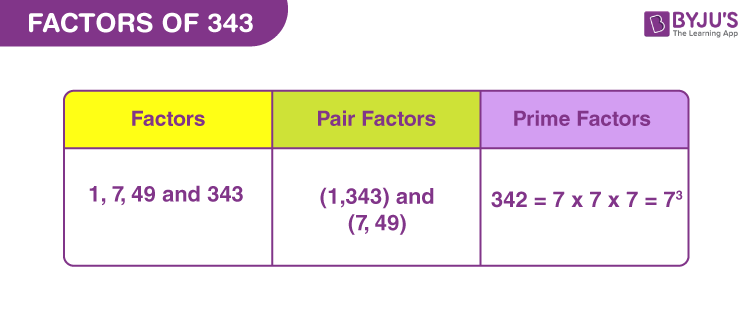# Factors of 343

Factors of 343 are the integers that divide the original number (i.e. 343) completely. Since 343 is a composite number, it will have more than 2 factors. Also, 343 is a perfect cube, such that;

343 = 7 x 7 x 7 = 73

By this, we can conclude that 7 is one of the factors of 343. To check, we can divide 343 by 7.

343 ÷ 7 = 49

Thus, we get a whole number after division. Hence, 7 divides 343 into 49 equal parts.

Now let us learn to find the other factors along with pair factors and prime factors.## How to Find Factors of 343?

Factors of 343 are the numerical values that divide the original number, without leaving any remainder. Therefore, dividing 343 by the smallest natural number, 1, will result in the actual number. Since 343 is an odd number, it cannot be divided by 2 or any even numbers.

343 ÷ 1 = 343

343 ÷ 7 = 49

343 ÷ 49 = 7

343 ÷ 343 = 1

Thus, we can conclude that only 1, 7, 49 and 343 are the factors of 343.

## Pair Factors of 343

To find the pair factors of 343, we need to find the product of the two numbers, to get the original number.

1 × 343 = 343

7 × 49 = 343

Therefore, the pair factors are (1, 343) and (7, 49). Similarly, we can consider negative pair factors of 343, since the product of two such negative factors will result in a positive number.

-1 × -343 = 343

-7 × -49 = 343

Therefore, the negative pair factors are (-1, -343) and (-7, -49).

## Prime Factorisation of 343

Prime factors are the prime numbers such as 2, 3, 5, 7, etc., that themselves have only two factors. Now to find the prime factors of a number, we need to check if the original number is completely divisible by that prime number or not. Thus, we will start dividing the original number by the smallest prime factor.

Step 1: Divide 343 by the smallest prime factor.

343/7 = 49

Step 2: Again divide 49 by the smallest prime factor.

49/7 = 7

Step 3: Again, divide 7 by 7.

7/7 = 1

Step 4: Since 1 is not divisible by any other factor apart from itself. Thus. we will stop our method here. Hence,

 Prime factorisation of 343 = 7 x 7 x 7 = 73

## Solved Examples

Q.1: If 343 copies are to be distributed among 49 students in a class. How many copies does each student get?

Solution: Given,

Number of copies = 343

Number of students in class = 49

Therefore, number of copies, each student will get = 343/49 = 7

Q.2: Find the sum of all the factors of 343.

Solution: The factors of 343 are 1, 7, 49 and 343.

Sum = 1+7+49+343 = 400

Therefore, 400 is the required sum.

Q.3: What are the common factors of 49 and 343?

Answer: Both 49 and 343 are composite numbers. Thus, their factors are:

49 → 1, 7 and 49

343 → 1, 7, 49 and 343

As we can see, 343 has all the factors of 49. Therefore, 1, 7 and 49 are the common factors for 49 and 343.

## Frequently Asked Questions on Factors of 343

### 1. How many factors of 343 are there?

Answer: There are four factors of 343. They are 1, 7, 49 and 343.

### 2. What two factors make 343?

Answer: When we multiply 7 by 49, we get 343. Thus 7 and 49 are two such factors, whose product gives the original number.

### 3. Is 343 a prime number?

Answer: 343 is not a prime number but a composite number, since it has more than two factors.

### 4. Is 343 a perfect square?

Answer: 343 is a perfect cube and not a perfect square, such that;

343 = 7 x 7 x 7 = 73

### 5. Write the first five multiples of 343.

Answer: Multiples can be obtained by multiplying 343 to the natural numbers. Hence, the first five multiples of 343 are:

343 x 1 = 343

343 x 2 = 686

343 x 3 = 1029

343 x 4 = 1372

343 x 5 = 1715

### 6. What are the prime factors of 343?

Answer: The prime factorisation of 343 is equal 7 x 7 x 7 = 73. Therefore, 7 is the prime factor of 343.